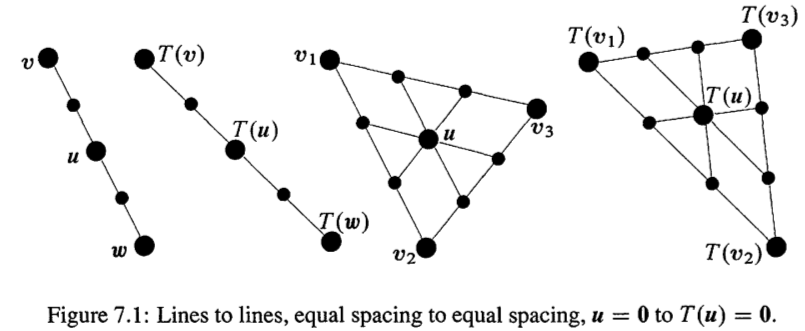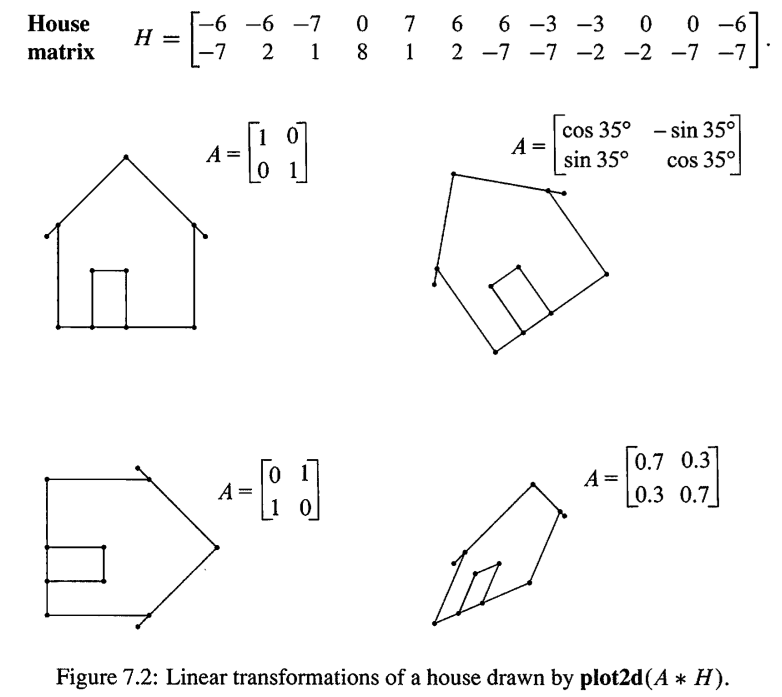Abstract: 本篇介绍线性代数的另一个角度，就是线性变换思想
Keywords: Linear Transformation，Linear Combination，Kernel，Range

# 线性变换思想

## 线性变换思想(The Idea of a Linear Transformation)

$$T(x+y)=T(x)+T(y)\\ T(\alpha x)=\alpha T(x)$$

$$T(\alpha x + \beta y)=\alpha T(x) + \beta T(y)$$

A Transformation T assigns an output $T(v)$ to each input vector $v$ in $V$. The transformation is linear if it meets these requirement for all $\vec{v}$ and $\vec{w}$:
$$T(v+w)=T(v)+T(w)\\ T(cv)=cT(v)$$
or take the two equation into one:
$$T(cv+dw)=cT(v)+dT(w)$$

$$T(v)=Av+u_0$$

$$T(v+w)=Av+Aw+u_0 \neq Av+u_0+Aw+u_0=T(v)+T(w)$$

## 线到线，三角到三角(Lines to Lines,Triangles to Triangles)

$$c’=\frac{1}{2}T(a)+\frac{1}{2}T(b)=T(\frac{1}{2}a+\frac{1}{2}b)=T(c)$$$$u=c_1v_1+c_2v_2+\dots + c_nv_n\\ T(u)=c_1T(v_1)+c_2T(v_2)+ \dots + c_nT(v_n)$$

。对应的就是nullspace和columnspace不过我们只叫他们kernel或者range，因为我们还没给出矩阵的定义，下篇才会给出。

## 平面线性变换(Linear Transformation of the Plane)0%